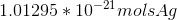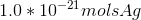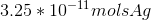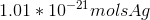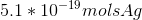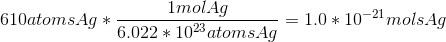# AP Chemistry : Atomic Structure and Properties

## Example Questions

### Example Question #16 : Measurements

What is the mass of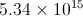particles of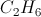?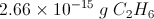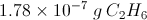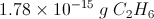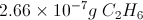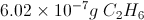Explanation: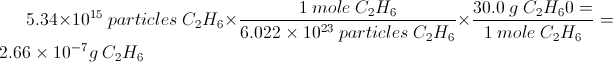### Example Question #1 : Using Avogadro's Number

How many atoms are in 1 mole of H2?

1.2044 * 1024

6.022 * 1046

3.626 * 1047

6.022 * 1023

1.2044 * 1024

Explanation:

This question requires an understanding of what avogadro's number actually represents.  Avogadro's number, 6.022 * 1023 is the number of things in one mole.  The question indicates that there is 1 mole of H2.  Thus there are 6.022 * 1023 molecules of H2.  However the question is asking for the amount of atoms in 1 mole of H2.  Thus we must consider the makeup of an H2 molecule, where we see that it is a diatomic molecule.  Thus we must multiply 6.022 * 1023 by 2 to calculate the number of individual atoms present in 1 mole of H2.  We find our answer to be 1.2044 * 1024.

### Example Question #18 : Measurements

A chemist has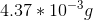of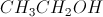.

How many molecules ofdoes she have?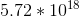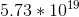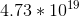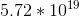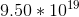Explanation: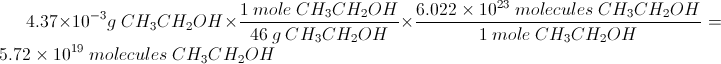### Example Question #2 : Using Avogadro's Number

How many hydrogen atoms are in 46.3 g of ethanol,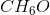?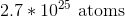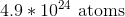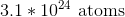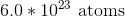Explanation:

To determine the number of hydrogen atoms, divide the mass of ethanol by its molar mass to get moles of ethanol.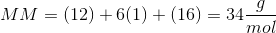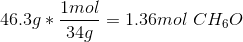Multiply this by six atoms of hydrogen per molecule of ethanol and by Avogadro's number to get the number of hydrogen atoms.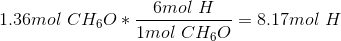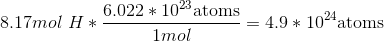### Example Question #20 : Measurements

How many hydrogen atoms are present in 500 mL of water at room temperature?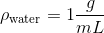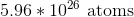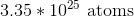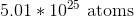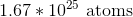Explanation:

Use the density of water, the molar mass of water, and Avogadro's number to calculate the number of molecules of water.

We have 500mL of water. Use the density to convert this to grams; then use the molar mass of water to convert this to moles.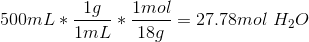There are two moles of hydrogen atoms per one mole of water.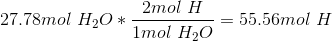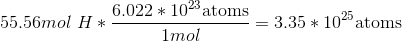### Example Question #1 : Moles And Molar Mass

Avogadro's number can convert all of the following units into moles, and vice versa, except __________.

Atoms

Particles

Molecules

Formula units

Grams

Grams

Explanation:

Avogadro's number can change: atoms, molecules, particles, formula units, and photons into moles (and vice versa). But in order to change grams to moles or moles to grams, the molar mass is needed.

### Example Question #2 : Moles And Molar Mass

A ring of imitation jewelry is coated with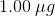of silver which is equivalent to: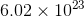atoms of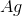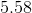atoms of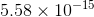atoms of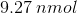ofor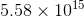atoms ofoforatoms ofExplanation:

To solve this problem we use the concept of atomic mass and Avogadro's number: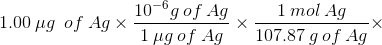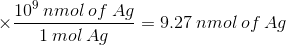And the number of Ag atoms will be: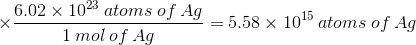### Example Question #1 : Moles And Molar Mass

Nuclear power has the potential to supply large amounts of energy from a relatively small amount of starting material (when compared to combustion of fossil fuels). Nuclear fuels have different yields of energy per unit mass (as well as radioactive wastes). For the following problem assume the following:

1 kilowatt hour =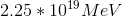United states energy consumption per capita per year = 13246.00kWH

The energy released in the nuclear fission of one atom of uranium-235 is 211.30MeV.

The natural abundance of uranium-235 is 0.72%

The energy released from the fission of one atom of thorium-232 is 191.00MeV (Involves several steps known as the thorium fuel cycle).

Thorium-232 is the most abundant isotope of thorium and for this problem can be considered to be 100%

Assume that 100% of the U-235 and Th-232 can be converted into energy.

How many grams of thorium or uranium (of all isotopes mined from natural sources) must be mined to provide the energy consumption needs of one average American in a year (given the above assumptions)?

Note: solve this problem twice; assuming 100% of energy consumption is produced from thorium in the first case and uranium in the second.

Thorium =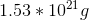Uranium =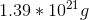Thorium =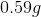Uranium =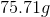Thorium =Uranium =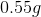Thorium =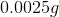Uranium =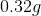Thorium =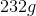Uranium =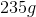Thorium =Uranium =Explanation:

To solve this problem we need to do some stoichiometry. Once we have gathered all the conversion information needed it becomes a matter of stringing the equation together and making sure our units cancel out to give us the units desired. Trying to tackle this problem stepwise could soon become confusing as there are more than a few steps involved.

## Calculation for the Mass of Uranium Mined to Supply the Energy Needs of 1 American for a Year: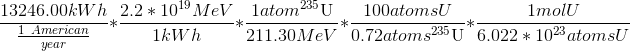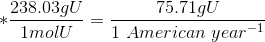It is worth noting that this is the mass of U and doesn't account for the fact that the U mined is not present as a pure element and therefore in reality the mass of material mined overall to get that 75.71g is much greater.

## Calculation for the mass of Thorium Mined to Supply the Energy Needs of 1 American for a Year: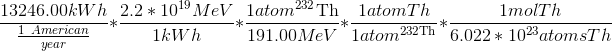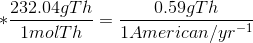The mass of Thorium mined is much less than the mass of Uranium mined mainly because of the concept of isotopic abundance. The isotope of thorium that is useful as nuclear fuel accounts for practically 100% of the naturally occurring thorium, whereas the useful isotope of uranium accounts for only 0.72% of the naturally occurring uranium. Also it is worth noting that like uranium, naturally occurring thorium is not present as a pure compound so therefore in reality the mass of material mined to get that 0.59g is also much greater. However it is also worth noting that thorium is much more abundant than uranium.

### Example Question #4 : Moles And Molar Mass

How many molecules are there in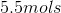of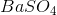?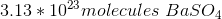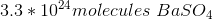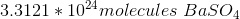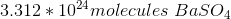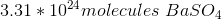Explanation:

Use dimensional analysis to convert from moles to molecules.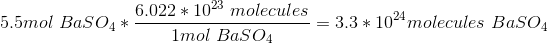Since the given measurement ofhas two significant figures, the answer must also have two significant figures.

### Example Question #5 : Moles And Molar Mass

How many moles are in 610 atoms of silver?